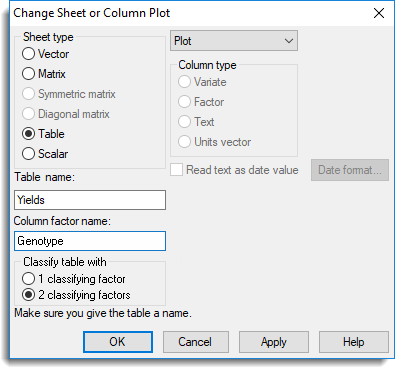1. Home
2. Converting a Vector Spreadsheet or a Column to a Different Type

# Converting a Vector Spreadsheet or a Column to a Different Type

A Vector spreadsheet containing variates, texts or factors can be converted to other types of spreadsheet such as a Table or Matrix. You can convert the entire sheet or select one or more columns to convert to different data types e.g. text to variate.

Note: This topic describes how to convert a Vector spreadsheet to another type. To convert other types of spreadsheet please refer to Converting a Spreadsheet Type.

• To change a column type refer to Converting a Column Type.2. Select a Sheet type. (Refer to Sheet Type in the Options list below for restrictions on spreadsheet conversions)
3. Additional fields may appear depending on which Sheet type you select. Click OK after setting additional options as described below.
Options

 Sheet type Converts the spreadsheet from one type to another. The following restrictions apply to spreadsheet conversion: A vector spreadsheet containing text columns cannot be converted to any other type. Converting a spreadsheet to type Scalar will preserve row 1 and discard all other rows. Spreadsheets can only be converted to diagonal or symmetric matrix type if they are square, i.e. have equal numbers of rows and columns Matrix name or Table name Change the name of matrices or tables by typing the new name into this field. Column factor name Specify the name of the factor indexing the columns within a two-way table. Classify table with When converting to a table, you can specify whether the new sheet has: 1 classifying factor – multiple one-way tables (one table per column). 2 classifying factors – a single two-way table with a factor indexing the columns.

Updated on March 1, 2019